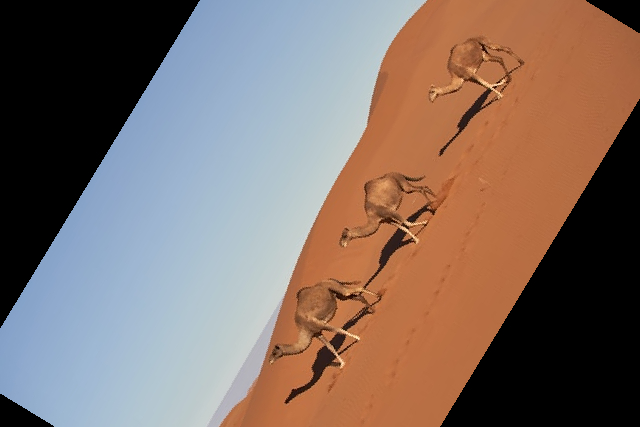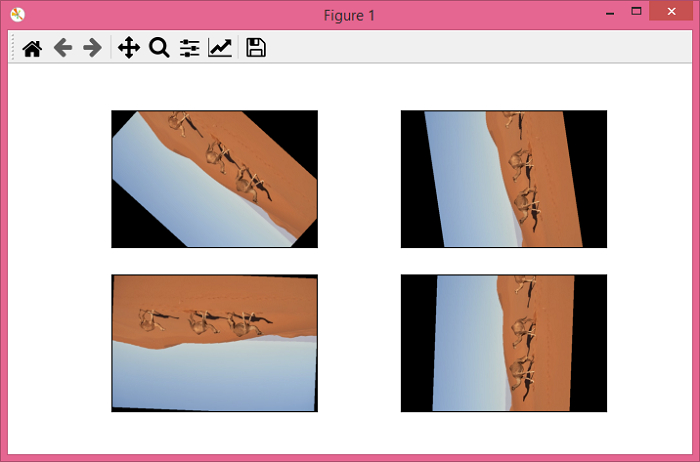# PyTorch – How to rotate an image by an angle?

PyTorchServer Side ProgrammingProgramming

RandomRotation() rotates an image by a random angle. The chosen random angle is from a given range of angles in degree. RandomRotation() is one of the many important transforms provided by the torchvision.transforms module. RandomRotation() transform accepts both PIL and tensor images.

A tensor image is a Torch tensor with shape [C, H, W], where C is the number of channels, H is the image height, and W is the image width. If the image is neither a PIL image nor a tensor image, then we first convert it to a tensor image and then apply the transform.

## Syntax

torchvision.transforms.RandomRotation(degree)(img)

Where degree is the desired range of degree. It's a sequence like (min, max). The image is rotated with a random angle selected from this range.

## Steps

We could use the following steps to rotate an image with a random angle −

• Import the required libraries. In all the following examples, the required Python libraries are torch, Pillow, and torchvision. Make sure you have already installed them.

import torch
import torchvision
import torchvision.transforms as T
from PIL import Image
• Read the input image. The input image is a PIL image or a torch tensor

img = Image.open('desert.jpg')
• Define a transform to rotate an image with a random angle. Give the desired range of degrees.

transform = T.RandomRotation((30,70))
• Apply the above-defined transform on the input image to rotate the input image with a random angle.

rotated_img = transform(img)
• Show the output image.

rotated_img.show()

## Input Image

This image is used as the input file in all the following examples.## Example 1

In this example, we set the range of angles to (30,60). The angle by which the input image is rotated is randomly picked from this range.

# import required libraries
import torch
import torchvision.transforms as T
from PIL import Image

img = Image.open('desert.jpg')

# define a transform to rotate he input image
transform = T.RandomRotation(degrees=(30,60))

# rotate the input image using above defined trasnform
img = transform(img)

# dispaly the rotated image
img.show()

## Output

It will produce the following output −Notice that the input image is rotated by an angle from the range (30,60).

## Example 2

In this example, we will demonstrate how you can rotate an input image by different angles.

import torch
import torchvision.transforms as T
from PIL import Image
import matplotlib.pyplot as plt

img = Image.open('desert.jpg')

# define a transform to rotate the image
transform = T.RandomRotation(degrees = (0,180))

# save four output images applying the above transform
imgs = [transform(img) for _ in range(4)]
fig = plt.figure(figsize=(7,4))
rows, cols = 2,2
for j in range(0, len(imgs)):
plt.imshow(imgs[j])
plt.xticks([])
plt.yticks([])
plt.show()

## Output

It will produce the following output −Notice that all four output images are rotated by different angles. The angle range is set to (0,180). Every image is rotated by an angle within the range (0,180).